## Encoder-decoder

seq2seq模型跟RNN（包括LSTM，GRU）的区别是什么？

RNN能用来做词性标注，输入是一个长度为a的序列，输出也是一个长度为a的序列；RNN能用来分类，输出是一个长度固定或者不固定的序列，输出为一个label/class。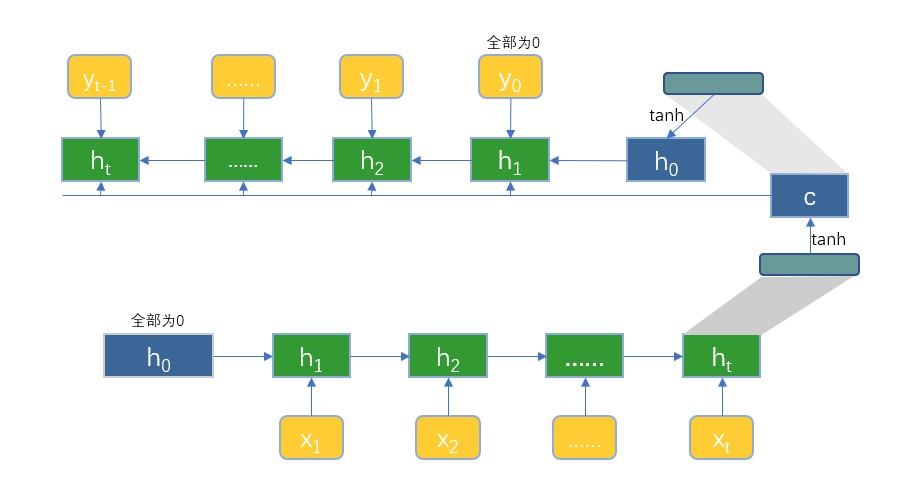decoder过程中，每一个hidden state，都是根据上一个hidden state，上一个输出$\hat y_{t-1}$，和encoder的context vector来计算的。那么，context vector是如何利用的呢？在传统的RNN中，都是根据上一个hidden state和当前的input（在这里是上一个输出）决定当前的hidden state，没有利用context vector这么一说。在LSTM中虽然引入了context，但是并不是一成不变的context，而是随着时间推移在不断学习变化的context。因此，encoder-decoder到底是如何将context vector纳入的，细节需要搞清楚！

#### encoder

$$h_i = (1- z_i) \odot h_{i-1} + z_i \odot \tilde h_i \\ \tilde h_i = \tanh (Wx_i + Ur_i \odot h_{i-1}) \\ z_i = \sigma(W_z x_i + U_z h_{i-1}) \\ r_i = \sigma(W_r x_i + U_r h_{i-1})$$

encoder由forward RNN和backward RNN组合而成，每一个时刻的hidden state由两个结果拼接而成。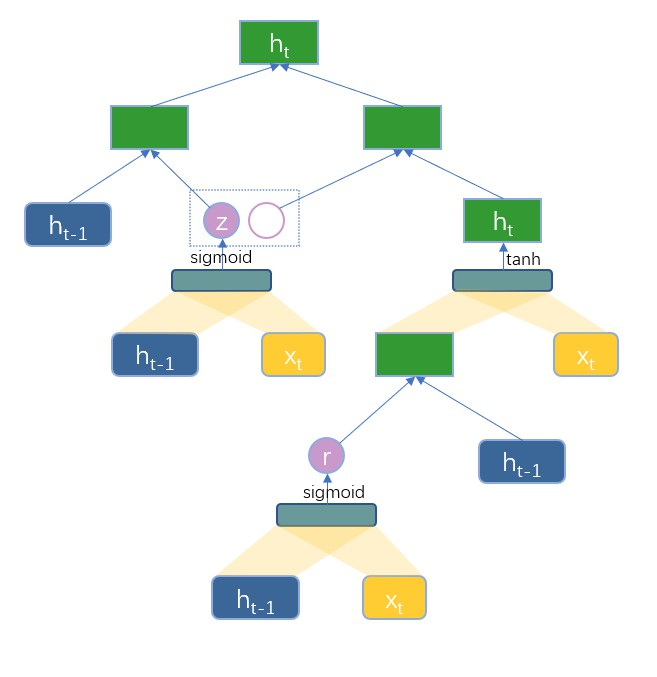#### decoder

$$s_i = (1-z_i) \odot s_i + z_i \odot \tilde s_i \\ \tilde s_i = \tanh (W y_{i-1} + U r_i \odot s_{i-1} + C c_i) \\ z_i = \sigma(W_z y_{i-1} + U_z s_{i-1} + C_z c_i) \\ r_i = \sigma(W_r y_{i-1} + U_r s_{i-1} + C_r c_i) \\$$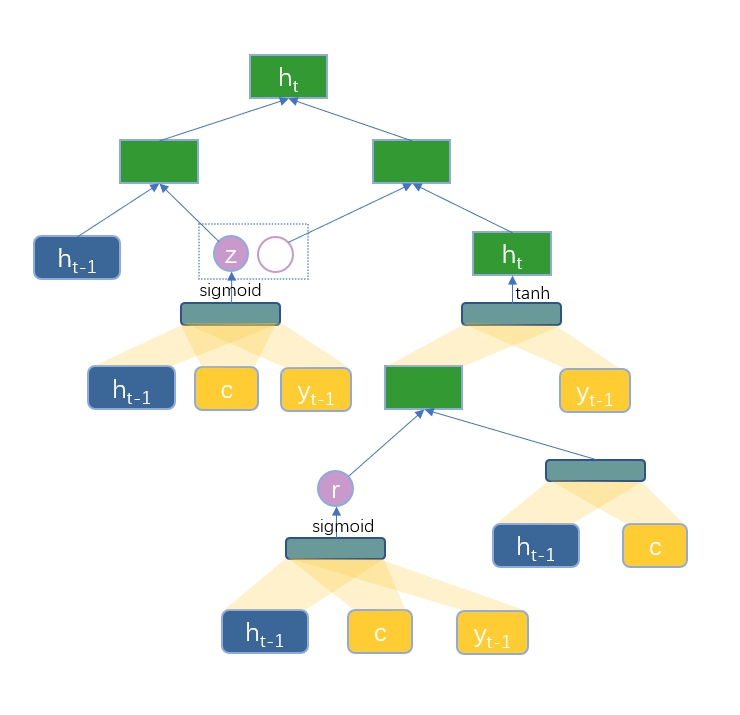### 预测

1. 首先，encoder按顺序处理source，最后生成一个定长的context vector。这个表示向量的实现方式是开放的，可以直接是encoder的最后的hidden state，也可以是最后的hidden state的函数，唯一确定的是，该向量的长度是固定的。
2. decoder在定长的context vector的基础上生成target sequence。在用RNN语言模型随机生成文本的时候，hidden state $h_t$是 $h_{t-1}$和上一个预测$\hat y_{t-1}$的函数，$\hat y_t$是$h_t$的函数；在这里，$h_t$和$\hat y_t$都跟上一个预测和context vector有关：

$$h_t = f(h_{t-1}, y_{t-1}, c) \\ y_t = g(h_t, y_{t-1}, c)$$

$$p(y_j| y_1^{t-1}, x_1^n) = \frac{\exp(y_j s_t)} {\sum_{j=1}^K \exp(y_js_t)} \\ s_t = O_h h_t + O_y y_{t-1} + O_c c$$

$$\frac{1}{m} \sum_{i=1}^m \log p(y_i|y_1^{i-1}, x_1^n)$$

## Attention

### 首次提出attention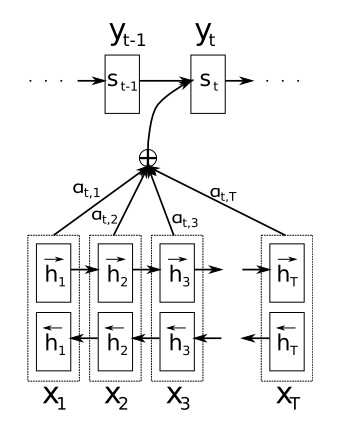1. RNN结构同样用的是GRU，但是encoder用的是Bi-RNN，这样的优势是能获得前向和后向依赖信息
2. decoder用到的context vector不再是一个固定的定长向量，而是动态生成的向量

### attention

$$c_i = \sum_{j=1}^{T_x} a_{ij}h_j$$

$$a_{ij} = \frac {\exp (e_{ij})} {\sum_{k=1}^{T_x} e_{ik}} \\ e_{ij} = v_a^T \tanh (W_a s_{i-1} + U_a h_j)$$

### Attention分类

content-based $\cos(s_t, h_j)$ 跟隐状态和$h_j$的内容有关，取二者的余弦相似度
additive/concatenation $v_a^T \tanh(W_a\cdot [s_t:h_j])$ 将二者拼接，然后通过一个feedword网络
location-based $softmax (W_a\cdot s_t)$ 只跟当前隐状态有关，隐状态中包含位置信息
General $s_t^TW_ah_j$ （之所以叫general，是因为能模拟大部分的关系？）
dot-product $s_t^Th_j$
scaled dot-product $\frac{s_t^Th_j}{\sqrt n}$

Inspired by reference

### Transformer

1. key, value, query分别该如何解读，代表什么呢？（已解答）
2. 具体的网络结构是咋样的？如何用encoder-decoder的思路来分析它？
3. 为什么只用self-attention就可以完成所有工作呢？
5. dot-product求得的attention的weights，好像没有经过softmax归一化？（有！！！不瞎的话你应该早就看到了！！！）
6. 多头self-attention如果都是同样的query, key, value的话，那么经过attention得到的应该都是同样的sequence of representation啊？如果都是一样的话，那还怎么学习不同的subspace的表示呢？难道只靠后面的feedword吗（feedforward不一样，它的weights的初始化的随机性，能保证学习到不一样的weights）？大概是因为在由embedding+position到进行attention的过程中，有一个线性变换的过程，即乘以一个参数矩阵，使得输出self-attention的dimension匹配一致。
7. 在decoder过程中，为避免leftward的影响，即当前word之后的words对预测的影响，使用了mask attention，具体是怎么实现的呢？（将当前单词后面的embedding设置为$- \infty$，如此一来，用当前word的embedding去跟$-\infty$进行attention的时候，内积为负无穷，经softmax的幂指数处理为0，即不参与attention。）
8. positional encoding是怎么做的？（目前还没看明白那两个公式是怎么应用的！！！
9. 边界情况：decoder的第一个单词的预测是怎么进行的？是否需要给每句话加上开始和结束的token呢？

#### 网络结构-encoder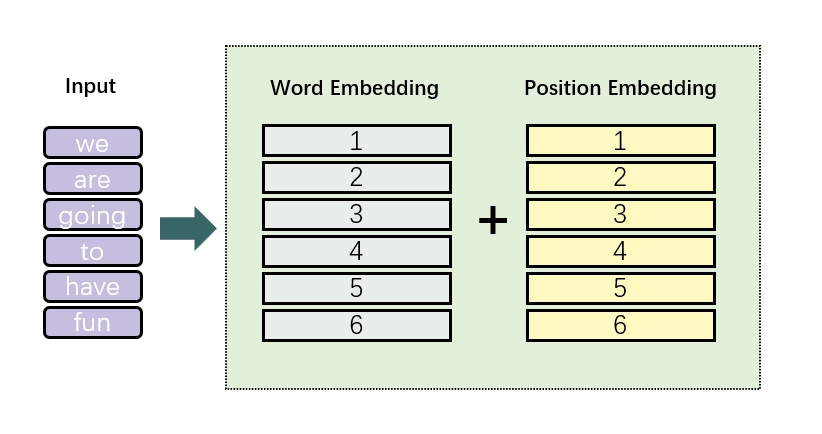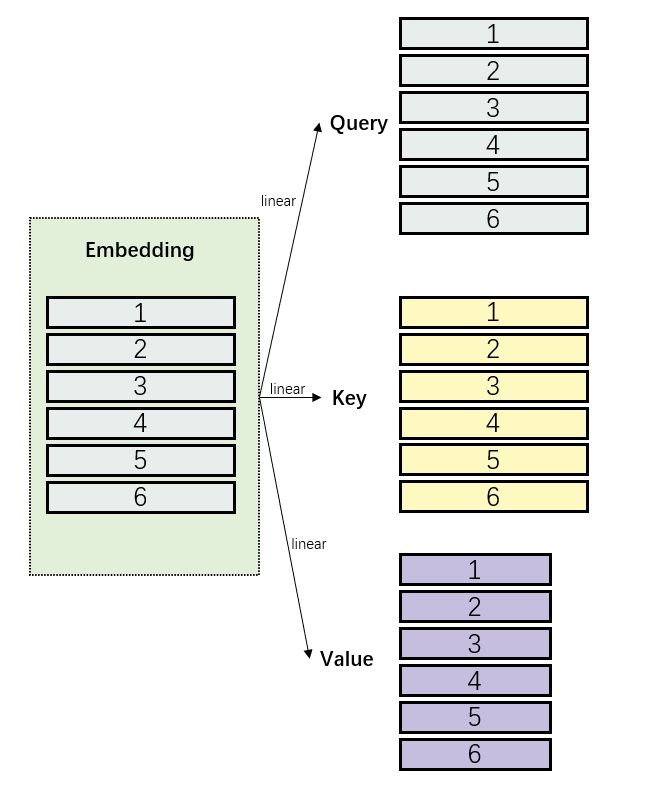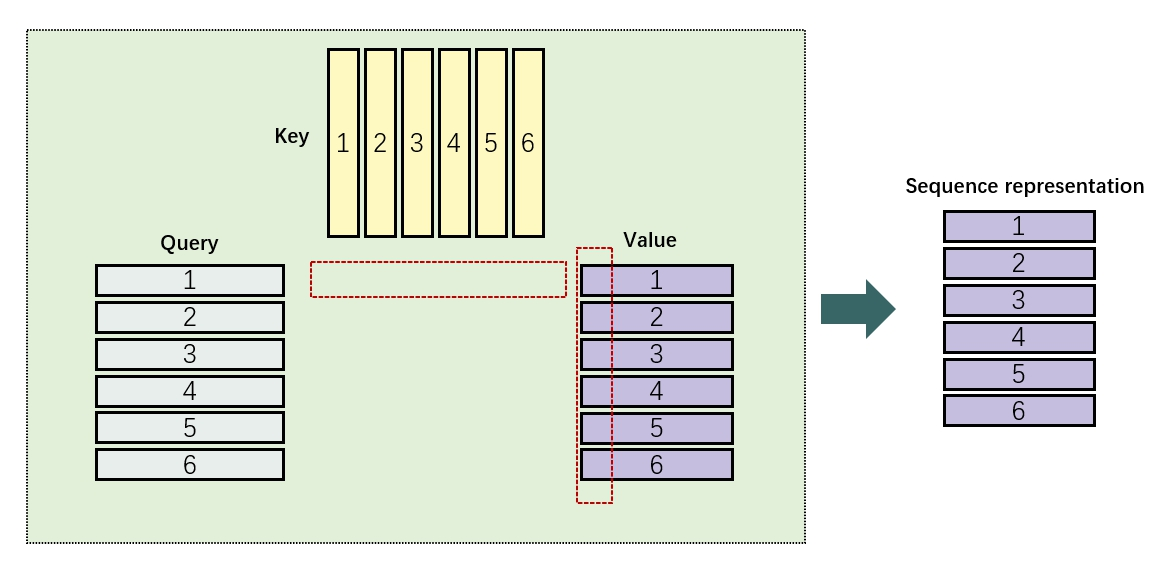$$a(Q,K, V) = softmax(\frac{QK^T}{\sqrt d_k})V$$
$d_k$是query的维度（Q为$l\times d_k$，$l$是input length），左边进过softmax得到的就是上图中中间部分的matrix，然后乘以$V$，便得到了最终的序列表征。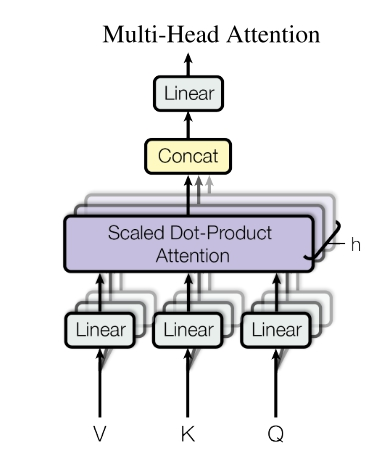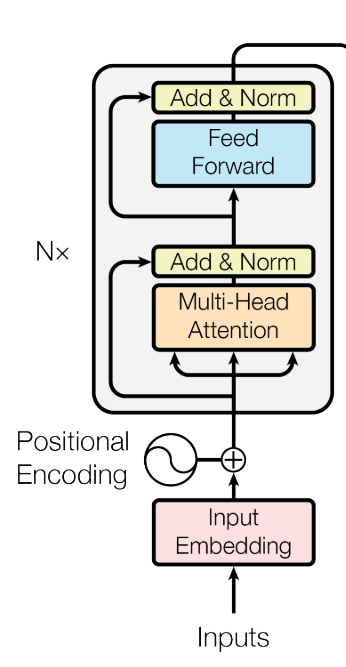#### 网络结构-decoder

decoder总体可以分为三个sublayer，第一个是self-attention，第二个是encoder-decoder attention，第三个是position-wise前馈神经网络。

## Reference

https://lilianweng.github.io/lil-log/2018/06/24/attention-attention.html#whats-wrong-with-seq2seq-model